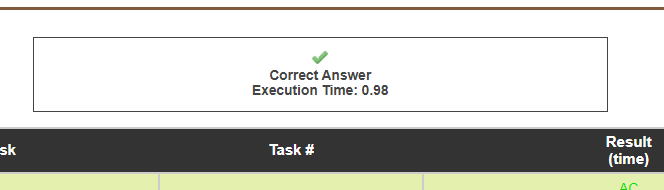# Very weak test cases in Bamboo Art (ZCO16002)

I was solving this problem and got 100 points.

This submission ran in 0.98s as seen below. I sorted the vector, considered every pair `a[i], a[j]` with `i < j`. Then using their common difference, I checked if the next value exists with the help of binary search (basic Arithmetic progression).Since this is very slow (1 second time limit), I decided to try to use pragmas to decrease runtime. I don’t know much about that so I failed to get 100 points. I decided to submit my code without pragmas (the original submission). It ran in 0.99s.I don’t know why the exact same submission ran slower. Can someone please explain this?
For the most important part, I was annoyed about my code being slow. I decided to use `unordered_map`. I began to doubt the test cases. My solution with `unordered_map` got accepted in 0.36s which is almost 3x faster.
I blew up `unordered_map`.
I created an input file with 2500 occurrences of 99733 using this simple python code:

``````f = open("test.txt", "a")
s = "2500 "
for i in range(2500):
s += "99733 "
f.write(s)
f.close()
``````

The same solution which ran in 0.36s is running since 10 minutes.

``````#include <bits/stdc++.h>
#include <ext/pb_ds/assoc_container.hpp>
#include <ext/pb_ds/tree_policy.hpp>

#define ll long long

using namespace __gnu_pbds;
using namespace std;

template <class T> using oset = tree <T, null_type, less <T>, rb_tree_tag, tree_order_statistics_node_update>;

void usaco(string name = "")
{
ios_base::sync_with_stdio(0);
cin.tie(0);
cout.tie(0);
if(name.size())
{
freopen((name+".txt").c_str(), "r", stdin);
freopen((name+".out").c_str(), "w", stdout);
}
}

int main()
{
usaco("test");
int n, ans = 1;
cin >> n;
vector <int> a(n);
unordered_map <int, int> m;
for (int i = 0; i < n; ++i) cin >> a[i], ++m[a[i]];
sort(begin(a), end(a));
for (int i = 0; i < n-1; ++i)
{
for (int j = i+1; j < n; ++j)
{
int d = a[j]-a[i];
int cur = a[j]+d;
int t = 2;
while (m[cur])
{
++t;
cur += d;
}
ans = max(ans, t);
}
}
cout << ans << '\n';
}
``````

This shows how bad the test cases are. Anything above O(n^2) should not pass according to the constraints. Is it possible for the solutions to be rejudged?
Also, does anyone know how to do it in O(n^2)?1 Like

replacing unordered map with an array can reduce it to n^2
Smthng like bool mp[mx+1]

1 Like

Since mp takes O(1) final complexity will be O(n^2)